# Ch10_Power Point.ppt

28. May 2023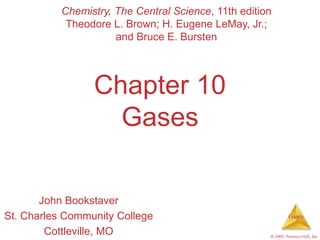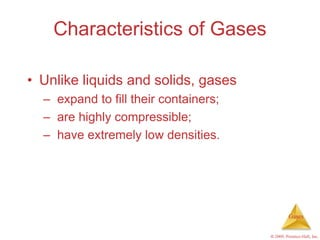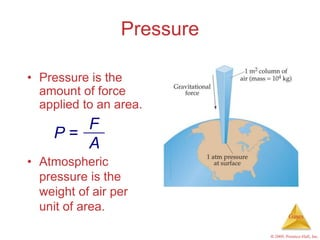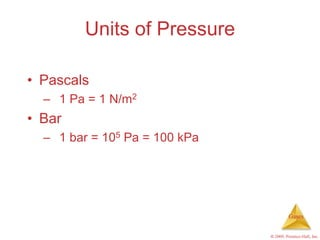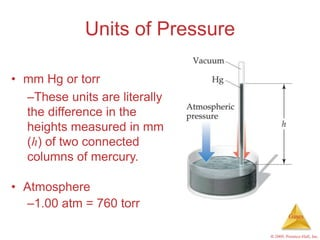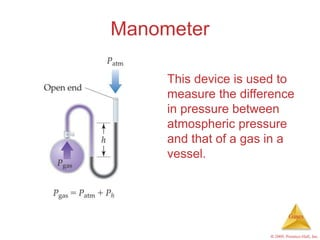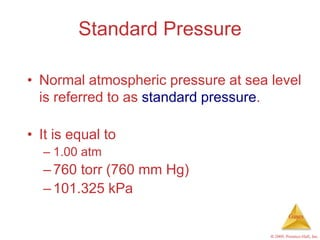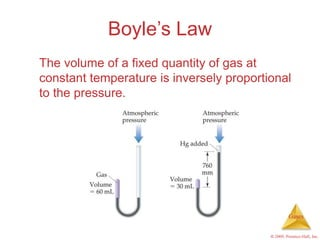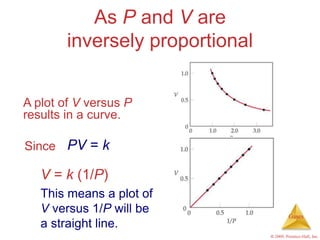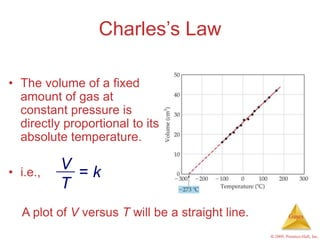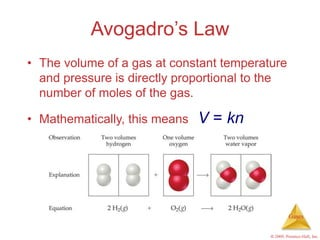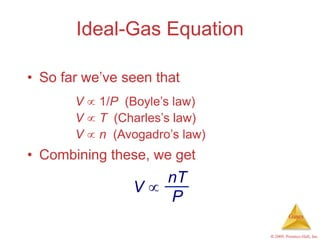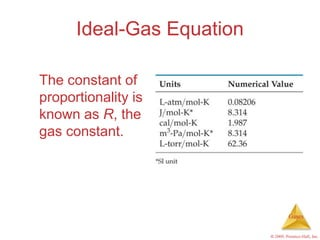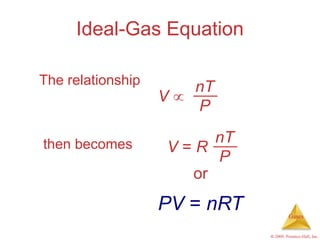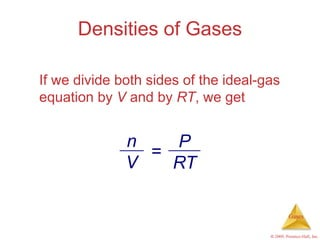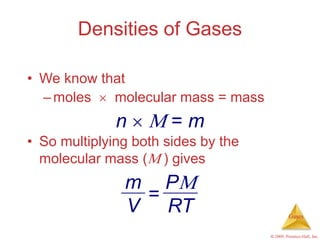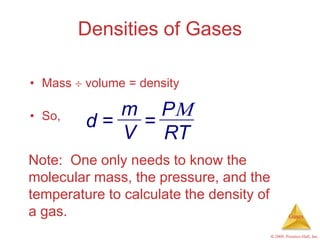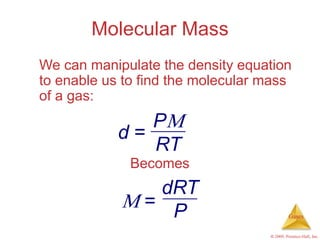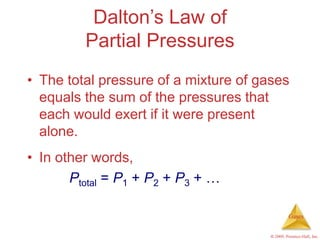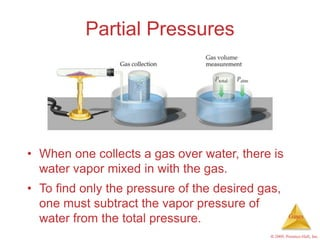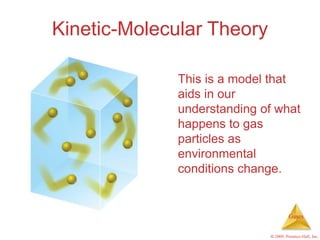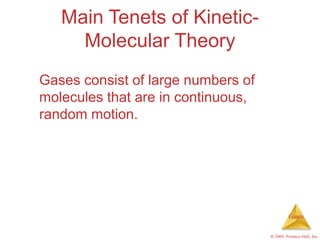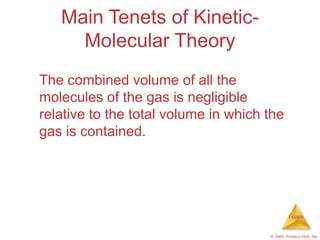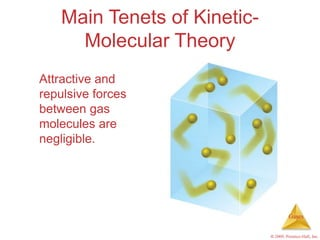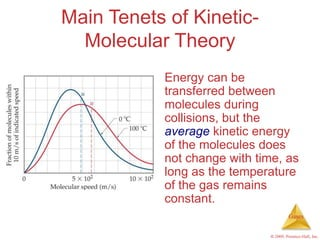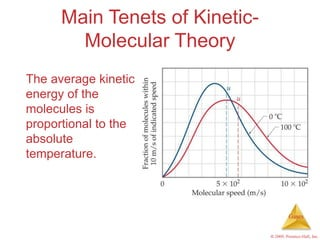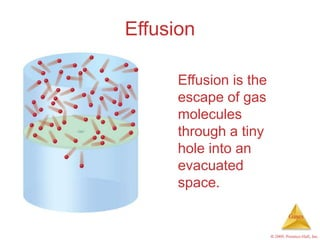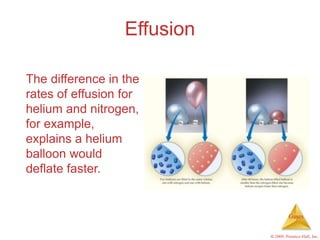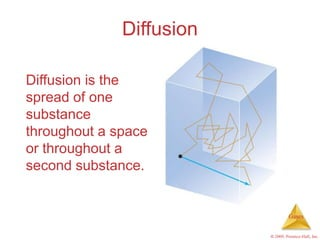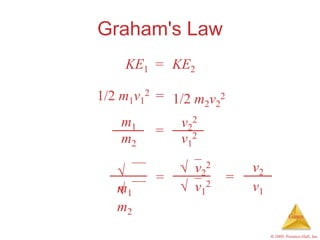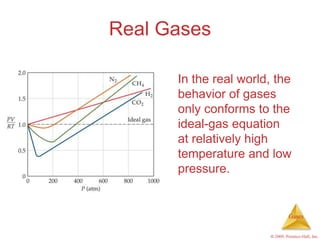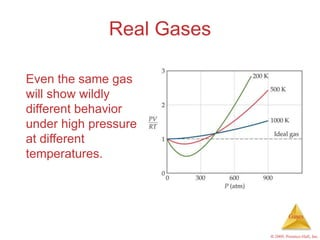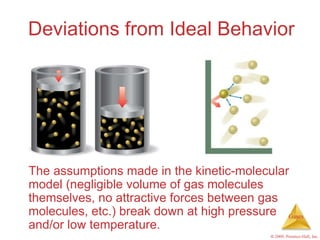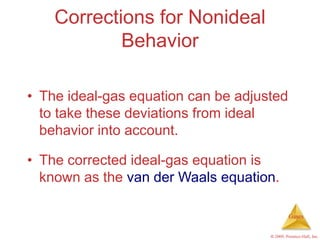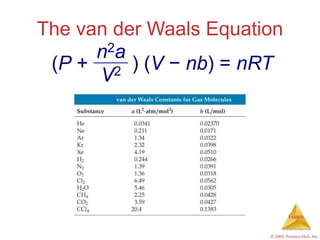1 von 35

### Ch10_Power Point.ppt

• 1. Gases © 2009, Prentice-Hall, Inc. Chapter 10 Gases John Bookstaver St. Charles Community College Cottleville, MO Chemistry, The Central Science, 11th edition Theodore L. Brown; H. Eugene LeMay, Jr.; and Bruce E. Bursten
• 2. Gases © 2009, Prentice-Hall, Inc. Characteristics of Gases • Unlike liquids and solids, gases – expand to fill their containers; – are highly compressible; – have extremely low densities.
• 3. Gases © 2009, Prentice-Hall, Inc. • Pressure is the amount of force applied to an area. Pressure • Atmospheric pressure is the weight of air per unit of area. P = F A
• 4. Gases © 2009, Prentice-Hall, Inc. Units of Pressure • Pascals – 1 Pa = 1 N/m2 • Bar – 1 bar = 105 Pa = 100 kPa
• 5. Gases © 2009, Prentice-Hall, Inc. Units of Pressure • mm Hg or torr –These units are literally the difference in the heights measured in mm (h) of two connected columns of mercury. • Atmosphere –1.00 atm = 760 torr
• 6. Gases © 2009, Prentice-Hall, Inc. Manometer This device is used to measure the difference in pressure between atmospheric pressure and that of a gas in a vessel.
• 7. Gases © 2009, Prentice-Hall, Inc. Standard Pressure • Normal atmospheric pressure at sea level is referred to as standard pressure. • It is equal to – 1.00 atm –760 torr (760 mm Hg) –101.325 kPa
• 8. Gases © 2009, Prentice-Hall, Inc. Boyle’s Law The volume of a fixed quantity of gas at constant temperature is inversely proportional to the pressure.
• 9. Gases © 2009, Prentice-Hall, Inc. As P and V are inversely proportional A plot of V versus P results in a curve. Since V = k (1/P) This means a plot of V versus 1/P will be a straight line. PV = k
• 10. Gases © 2009, Prentice-Hall, Inc. Charles’s Law • The volume of a fixed amount of gas at constant pressure is directly proportional to its absolute temperature. A plot of V versus T will be a straight line. • i.e., V T = k
• 11. Gases © 2009, Prentice-Hall, Inc. Avogadro’s Law • The volume of a gas at constant temperature and pressure is directly proportional to the number of moles of the gas. • Mathematically, this means V = kn
• 12. Gases © 2009, Prentice-Hall, Inc. Ideal-Gas Equation V  1/P (Boyle’s law) V  T (Charles’s law) V  n (Avogadro’s law) • So far we’ve seen that • Combining these, we get V  nT P
• 13. Gases © 2009, Prentice-Hall, Inc. Ideal-Gas Equation The constant of proportionality is known as R, the gas constant.
• 14. Gases © 2009, Prentice-Hall, Inc. Ideal-Gas Equation The relationship then becomes nT P V  nT P V = R or PV = nRT
• 15. Gases © 2009, Prentice-Hall, Inc. Densities of Gases If we divide both sides of the ideal-gas equation by V and by RT, we get n V P RT =
• 16. Gases © 2009, Prentice-Hall, Inc. • We know that –moles  molecular mass = mass Densities of Gases • So multiplying both sides by the molecular mass ( ) gives n   = m P RT m V =
• 17. Gases © 2009, Prentice-Hall, Inc. Densities of Gases • Mass  volume = density • So, Note: One only needs to know the molecular mass, the pressure, and the temperature to calculate the density of a gas. P RT m V = d =
• 18. Gases © 2009, Prentice-Hall, Inc. Molecular Mass We can manipulate the density equation to enable us to find the molecular mass of a gas: Becomes P RT d = dRT P  =
• 19. Gases © 2009, Prentice-Hall, Inc. Dalton’s Law of Partial Pressures • The total pressure of a mixture of gases equals the sum of the pressures that each would exert if it were present alone. • In other words, Ptotal = P1 + P2 + P3 + …
• 20. Gases © 2009, Prentice-Hall, Inc. Partial Pressures • When one collects a gas over water, there is water vapor mixed in with the gas. • To find only the pressure of the desired gas, one must subtract the vapor pressure of water from the total pressure.
• 21. Gases © 2009, Prentice-Hall, Inc. Kinetic-Molecular Theory This is a model that aids in our understanding of what happens to gas particles as environmental conditions change.
• 22. Gases © 2009, Prentice-Hall, Inc. Main Tenets of Kinetic- Molecular Theory Gases consist of large numbers of molecules that are in continuous, random motion.
• 23. Gases © 2009, Prentice-Hall, Inc. Main Tenets of Kinetic- Molecular Theory The combined volume of all the molecules of the gas is negligible relative to the total volume in which the gas is contained.
• 24. Gases © 2009, Prentice-Hall, Inc. Main Tenets of Kinetic- Molecular Theory Attractive and repulsive forces between gas molecules are negligible.
• 25. Gases © 2009, Prentice-Hall, Inc. Main Tenets of Kinetic- Molecular Theory Energy can be transferred between molecules during collisions, but the average kinetic energy of the molecules does not change with time, as long as the temperature of the gas remains constant.
• 26. Gases © 2009, Prentice-Hall, Inc. Main Tenets of Kinetic- Molecular Theory The average kinetic energy of the molecules is proportional to the absolute temperature.
• 27. Gases © 2009, Prentice-Hall, Inc. Effusion Effusion is the escape of gas molecules through a tiny hole into an evacuated space.
• 28. Gases © 2009, Prentice-Hall, Inc. Effusion The difference in the rates of effusion for helium and nitrogen, for example, explains a helium balloon would deflate faster.
• 29. Gases © 2009, Prentice-Hall, Inc. Diffusion Diffusion is the spread of one substance throughout a space or throughout a second substance.
• 30. Gases © 2009, Prentice-Hall, Inc. Graham's Law KE1 KE2 = 1/2 m1v1 2 1/2 m2v2 2 = = m1 m2 v2 2 v1 2  m1  m2 v2 2 v1 2 = v2 v1 =
• 31. Gases © 2009, Prentice-Hall, Inc. Real Gases In the real world, the behavior of gases only conforms to the ideal-gas equation at relatively high temperature and low pressure.
• 32. Gases © 2009, Prentice-Hall, Inc. Real Gases Even the same gas will show wildly different behavior under high pressure at different temperatures.
• 33. Gases © 2009, Prentice-Hall, Inc. Deviations from Ideal Behavior The assumptions made in the kinetic-molecular model (negligible volume of gas molecules themselves, no attractive forces between gas molecules, etc.) break down at high pressure and/or low temperature.
• 34. Gases © 2009, Prentice-Hall, Inc. Corrections for Nonideal Behavior • The ideal-gas equation can be adjusted to take these deviations from ideal behavior into account. • The corrected ideal-gas equation is known as the van der Waals equation.
• 35. Gases © 2009, Prentice-Hall, Inc. The van der Waals Equation ) (V − nb) = nRT n2a V2 (P +